模型

基本元素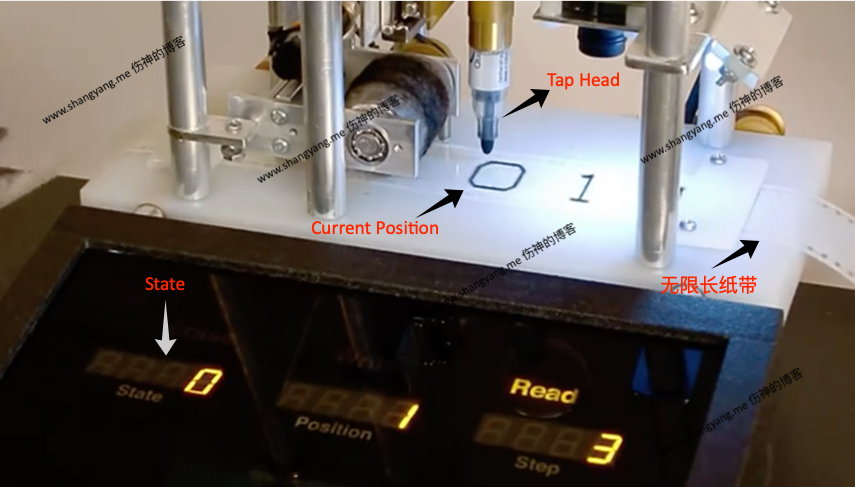1. 无限长的纸带(原文叫 Tape)，带有无限多个方格；且方格有个特性，就是可以被读写；
• 它始终指向当前的一个方格，当前方格的位置称作 current Position
• 它可以读取当前方格中的内容，该内容成为当前值 current Value；
• 它可以在当前方格中写入，如果当前方格已经有内容了，擦除后重写；
3. State，每一个 current Position 都有一个状态 State，它告诉当前的 Tap Head 该做什么，是对当前的方格进行只读或者读写？处理完以后，下一步是左移还是右移？
A State $\to$ A Rule，每一个 State 对应一个 Rule，正是这个 Rule 告诉 Tap Head 在当前位置该如何操作；

理论

有限状态机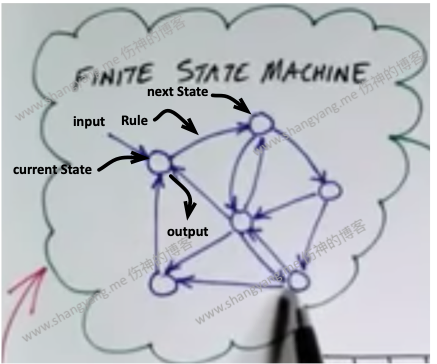• 当前状态 current State
• 输入
• 规则 Rule，在当前状态下执行的规则，该规则指定 next State；
• 输出
• 下一个状态 next State

图灵机

1. 状态
包含起始状态、中间状态和结束状态；起始状态用 $q_0$ 表示，结束状态用 $q_a$ 表示；
2. 输入
3. 输出
4. 移动
${L, R, N}$ 中的一个，其中 L 表示读写头向左移一格，R 表示读写头向右移一格，N 表示读写头不动

current State 输入值 输出值 移动 next State
$q_0$ 1 0 R $q_1$
$q_0$ 0 0 R $q_1$
$q_1$ 1 0 N $q_a$
$q_1$ 0 0 N $q_a$

• 从起始状态 $q_0$ 开始，
• 若当前值(输入值)为 1，则用 0 覆盖当前值，指针头右移一位，然后进入 $q_1$；
• 若当前值(输入值)为 0，则不变，指针头右移一位，然后进入 $q_1$；
• 进入中间状态 $q_1$，
• 若当前值(输入值)为 1，则用 0 覆盖当前值，指针头不动，最后进入结束状态 $q_a$，退出；
• 若当前值(输入值)为 0，则不变，指针头不动，最后进入结束状态 $q_a$，退出；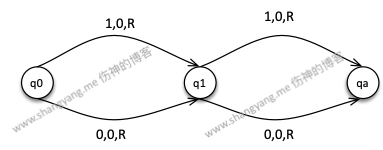实例

一进制加法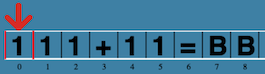1. 从起始位置开始，将 1 逐个移动到等号的右边，过程中清除+
2. 最后清除=
3. 剩下的部分便是最终的结果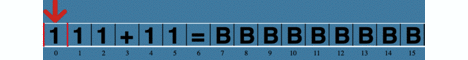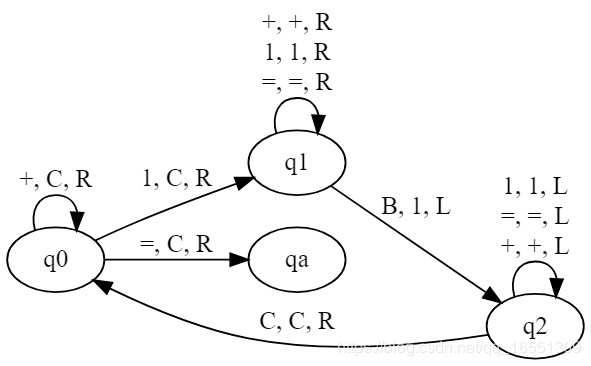二进制加法

• K表示当前位已经被处理过，
• a表示需要进入进位状态，比如 $1+1$，这个时候 $a=0$ 但是需要进入进位状态
• 进位状态，不断左移，如果遇到 0 则加为 1，若遇到1，则改写为0，继续进位；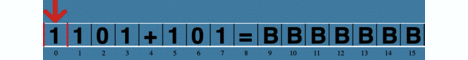不足

1. 一系列定义的规则只适合一种数学运算，图灵机的规则复杂且使用范围单一；
2. 没有保存运算中间结果；
3. 完美的图灵机其实是实现不了的，因为我们无法提供无限长的纸带条；

1. 提供了中间结果存储器，通过中间结果，使得可以同时完成相互依赖的多种数学运算；(这里的一种数学运算就等价于现代计算机的一个计算指令)
2. 并且将一种数学计算化简为一个计算机指令，比如一个加法指令(对比上述图灵机的加法，是不是简单了许多)，这样使得数学计算的设计更为简单；